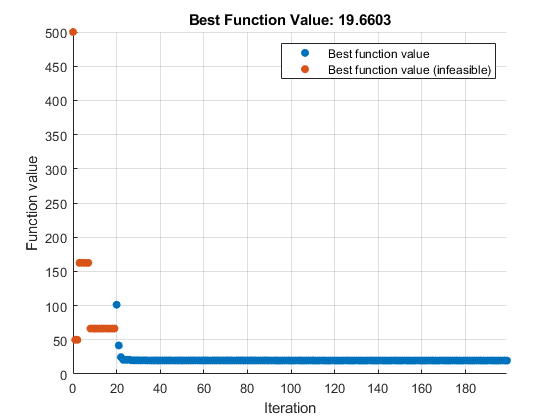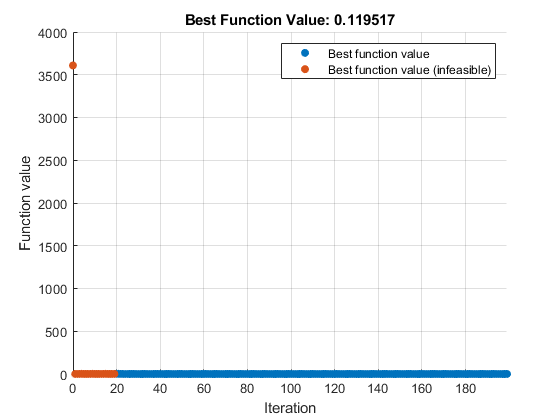## Convert Nonlinear Constraints Between `surrogateopt` Form and Other Solver Forms

### Why Convert Constraint Forms?

To try various solvers including `surrogateopt` on a problem that has nonlinear inequality constraints, you must convert between the form required by `surrogateopt` and the form required by other solvers.

### Convert from `surrogateopt` Structure Form to Other Solvers

The objective function `objconstr(x)` for `surrogateopt` returns a structure. The `Fval` field contains the objective function value, a scalar. The `Ineq` field contains a vector of constraint function values. The solver attempts to make all values in the `Ineq` field be less than or equal to zero. Positive values indicate a constraint violation.

Other solvers expect the objective function to return a scalar value, not a structure. Other solvers also expect the nonlinear constraint function to return two outputs, `c(x)` and `ceq(x)`, not a structure containing `c(x)`.

To convert the `surrogateopt` function `objconstr(x)` for use in other solvers:

• Set the objective function to `@(x)objconstr(x).Fval`.

• Set the nonlinear constraint function to `@(x)deal(objconstr(x).Ineq,[])`.

For example,

```function ff = objconstr(x) ff.Fval = norm(x)^2; ff.Ineq = norm(x - [5,8])^2 - 25; end```

To solve a constrained minimization problem using `objconstr`, call `surrogateopt`.

```lb = [-10,-20]; ub = [20,10]; sol = surrogateopt(@objconstr,lb,ub)```
```Surrogateopt stopped because it exceeded the function evaluation limit set by 'options.MaxFunctionEvaluations'. sol = 2.3325 3.7711```To solve the same problem using `fmincon`, split the objective and constraint into separate functions. Include the nonlinear equality constraint as `[]` by using the `deal` function.

```objfcn = @(x)objconstr(x).Fval; nlcon = @(x)deal(objconstr(x).Ineq,[]);```

Call `fmincon` with the objective function `objfcn` and nonlinear constraint function `nlcon`.

```[solf,fvalf,eflag,output] = ... fmincon(objfcn,[0,0],[],[],[],[],lb,ub,nlcon) ```
```Local minimum found that satisfies the constraints. Optimization completed because the objective function is non-decreasing in feasible directions, to within the value of the optimality tolerance, and constraints are satisfied to within the value of the constraint tolerance. solf = 2.3500 3.7600 fvalf = 19.6602 eflag = 1 output = struct with fields: iterations: 7 funcCount: 24 constrviolation: 0 stepsize: 2.0395e-05 algorithm: 'interior-point' firstorderopt: 4.9651e-06 cgiterations: 0 message: '↵Local minimum found that satisfies the constraints.↵↵Optimization completed because the objective function is non-decreasing in ↵feasible directions, to within the value of the optimality tolerance,↵and constraints are satisfied to within the value of the constraint tolerance.↵↵<stopping criteria details>↵↵Optimization completed: The relative first-order optimality measure, 6.602486e-07,↵is less than options.OptimalityTolerance = 1.000000e-06, and the relative maximum constraint↵violation, 0.000000e+00, is less than options.ConstraintTolerance = 1.000000e-06.↵↵' ```

You can also use `patternsearch` or `ga` to solve the problem using the same conversion.

### Convert from Other Solvers to `surrogateopt` Structure Form

If you have a problem written in the form for other solvers, use the `packfcn` function to convert the objective and nonlinear constraints to the structure form for `surrogateopt`. If the objective function is a function handle `@obj` and the nonlinear constraint function is `@nlconst`, then use the objective function `objconstr` for `surrogateopt`.

`objconstr = packfcn(@obj,@nlconst);`

In this example, the objective function is Rosenbrock's function.

```ros = @(x)100*(x(2) - x(1)^2)^2 + (1 - x(1))^2; ```

Specify the constraint function to restrict the solution to lie inside a disk of radius 1/3 centered at the point [1/3,1/3].

```function [c,ceq] = circlecon(x) c = (x(1)-1/3)^2 + (x(2)-1/3)^2 - (1/3)^2; ceq = [];```

Set bounds of –2 and 2 on each component.

```lb = [-2,-2]; ub = [2,2];```

Solve the problem using `patternsearch` starting from [0,0].

```x0 = [0,0]; x = patternsearch(ros,x0,[],[],[],[],lb,ub,@circlecon)```
```Optimization terminated: mesh size less than options.MeshTolerance and constraint violation is less than options.ConstraintTolerance. x = 0.6523 0.4258```

Convert the problem for solution by `surrogateopt`.

```objconstr = packfcn(ros,@circlecon); xs = surrogateopt(objconstr,lb,ub)```
```Surrogateopt stopped because it exceeded the function evaluation limit set by 'options.MaxFunctionEvaluations'. xs = 0.6543 0.4284```